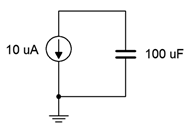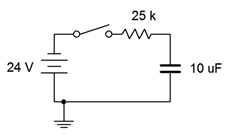# 6.1.4: Exercises

$$\newcommand{\vecs}{\overset { \rightharpoonup} {\mathbf{#1}} }$$ $$\newcommand{\vecd}{\overset{-\!-\!\rightharpoonup}{\vphantom{a}\smash {#1}}}$$$$\newcommand{\id}{\mathrm{id}}$$ $$\newcommand{\Span}{\mathrm{span}}$$ $$\newcommand{\kernel}{\mathrm{null}\,}$$ $$\newcommand{\range}{\mathrm{range}\,}$$ $$\newcommand{\RealPart}{\mathrm{Re}}$$ $$\newcommand{\ImaginaryPart}{\mathrm{Im}}$$ $$\newcommand{\Argument}{\mathrm{Arg}}$$ $$\newcommand{\norm}{\| #1 \|}$$ $$\newcommand{\inner}{\langle #1, #2 \rangle}$$ $$\newcommand{\Span}{\mathrm{span}}$$ $$\newcommand{\id}{\mathrm{id}}$$ $$\newcommand{\Span}{\mathrm{span}}$$ $$\newcommand{\kernel}{\mathrm{null}\,}$$ $$\newcommand{\range}{\mathrm{range}\,}$$ $$\newcommand{\RealPart}{\mathrm{Re}}$$ $$\newcommand{\ImaginaryPart}{\mathrm{Im}}$$ $$\newcommand{\Argument}{\mathrm{Arg}}$$ $$\newcommand{\norm}{\| #1 \|}$$ $$\newcommand{\inner}{\langle #1, #2 \rangle}$$ $$\newcommand{\Span}{\mathrm{span}}$$$$\newcommand{\AA}{\unicode[.8,0]{x212B}}$$

## Analysis

1. For the circuit shown in Figure 8.6.1 , determine the effective capacitance.Figure 8.6.1

2. Determine the effective capacitance of the configuration shown in Figure 8.6.2 .Figure 8.6.2

3. Given the capacitor network shown in Figure 8.6.3 , determine the effective value.Figure 8.6.3

4. Determine the effective capacitance of network shown in Figure 8.6.4 .Figure 8.6.4

5. Determine the voltage across each capacitor for the circuit shown in Figure 8.6.5 .Figure 8.6.5

6. Determine the voltage across each capacitor for the circuit shown in Figure 8.6.6 .Figure 8.6.6

7. Determine the initial voltage across each component for the circuit shown in Figure 8.6.7 .Figure 8.6.7

8. Given the network shown in Figure 8.6.7 , determine the steady-state voltage across each component.

9. For the circuit shown in Figure 8.6.8 , determine the capacitor voltage 3 microseconds after the power is switched on.Figure 8.6.8

10. For the circuit shown in Figure 8.6.9 , determine the capacitor voltage 5 seconds after the power is switched on.Figure 8.6.9

11. Determine the time constant and the time required to reach steady-state for the circuit shown in Figure 8.6.10 .Figure 8.6.10

12. For the circuit shown in Figure 8.6.10 , determine the capacitor voltage and circulating current 20 microseconds and 100 milliseconds after the switch is thrown.

13. Given the circuit shown in Figure 8.6.11 , determine the capacitor voltage and circulating current 200 milliseconds and 10 seconds after the switch is thrown.Figure 8.6.11

14. Determine the time constant and the time required to reach steady-state for the circuit shown in Figure 8.6.11 .

15. Determine the time constant and the time required to reach steady-state for the circuit shown in Figure 8.6.12 , switch position 1.Figure 8.6.12

16. Determine the charge and discharge time constants for the circuit shown in Figure 8.6.13 .Figure 8.6.13

17. Given the circuit shown in Figure 8.6.12 , determine the capacitor voltage 10 milliseconds after the power is turned on. At this point, the switch is thrown to position 2. Determine how long it will take the capacitor to discharge to nearly zero volts.

18. For the circuit shown in Figure 8.6.13 , determine the capacitor voltage 400 microseconds after the power is turned on. At this point, the switch is thrown to position 2. Determine how long it will take the capacitor to discharge to nearly zero volts.

19. Determine the time constant and the time required to reach steady-state for the circuit shown in Figure 8.6.14 , switch position 1.Figure 8.6.14

20. Given the circuit shown in Figure 8.6.14 , determine the capacitor voltage 12 milliseconds after the power is turned on. At this point, the switch is thrown to position 2. Determine how long it will take the capacitor to discharge to nearly zero volts.

## Design

21. Given the circuit of Figure 8.6.10 , determine a new resistor value such that steady-state is reached in 2 milliseconds.

22. Given the circuit of Figure 8.6.11 , determine a new resistor value such that steady-state is reached in 5 seconds.

23. When an audio amplifier is turned on, the power surge can cause an audible pop from the loudspeaker. To prevent this, amplifiers often connect to the loudspeaker via a relay. The relay is energized to connect the loudspeaker once the output has settled, typically a few seconds after power is applied. This delay may be created via an RC network. Suppose the driving circuit is 5 volts and the relay trips at 4 volts. Further, the associated charging resistance is 10 k$$\Omega$$. Determine the capacitance required to achieve a 2 second delay time.

## Challenge

24. Determine the time constant and the time required to reach steady-state for the circuit shown in Figure 8.6.15 .Figure 8.6.15

25. For the circuit shown in Figure 8.6.15 , determine the capacitor voltage 1 second after the power is turned on. At this point, the switch is thrown to position 2. Determine how long it will take the capacitor to discharge to nearly zero volts.

## Simulation

26. Perform a transient analysis to verify the time to steady-state of Figure 8.6.10 (problem 11).

27. Perform a transient analysis to verify the time to steady-state of Figure 8.6.11 (problem 14).

28. Use a transient analysis to verify the design of problem 21.

29. Use a transient analysis to verify the design of problem 22.

30. Use a transient analysis to verify the operation of the circuit shown in Figure 8.6.15 as specified in problem 25. You may wish to do this as two separate simulations, one for each switch position, with the second position using a pre-charged capacitor.

This page titled 6.1.4: Exercises is shared under a CC BY-NC-SA license and was authored, remixed, and/or curated by James M. Fiore.